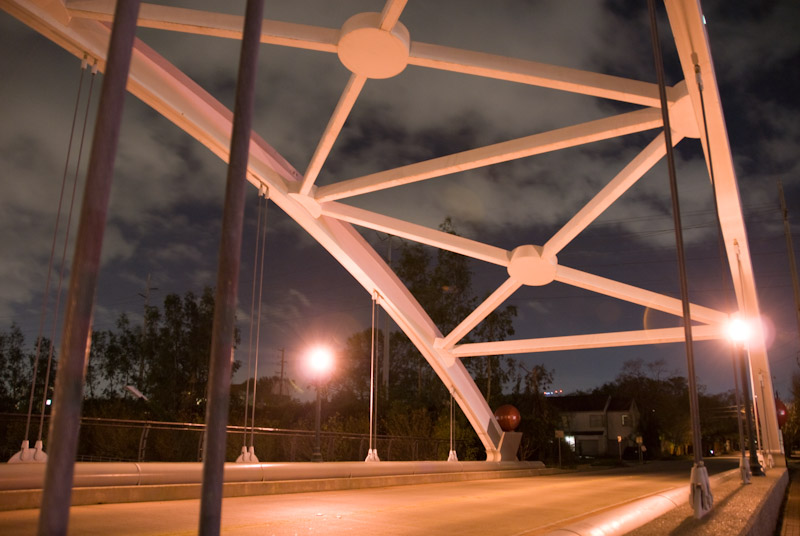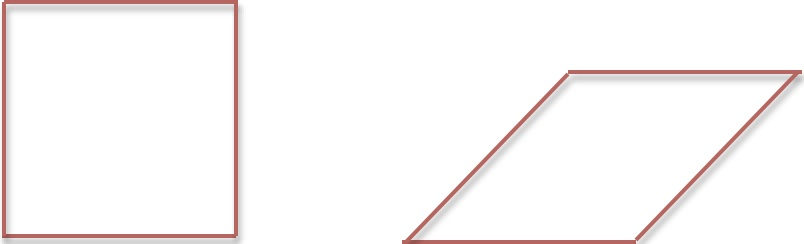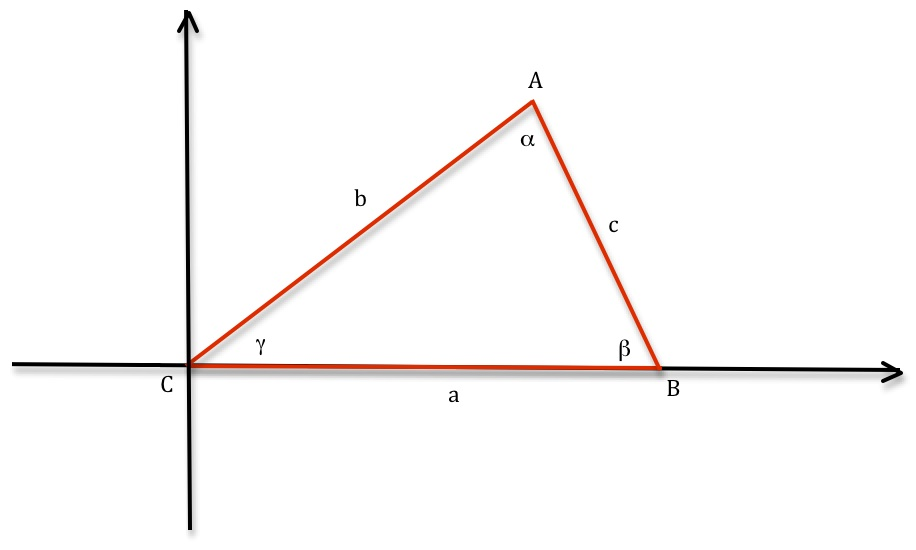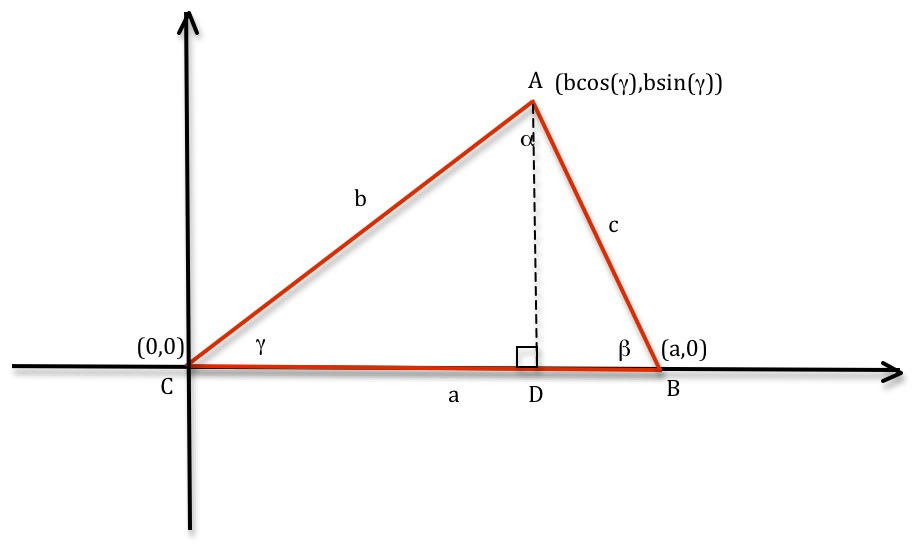# Why are there triangles in buildings and bridges?

Posted by: Gary Ernest Davis on: October 7, 2010

When you look around at the geometry of the built environment – buildings and bridges, among other structures – you see quite a few triangles:Why is this?

What is it about triangles that we see them in built structures? Do they look pretty, and are there for ornamentation? Are the architects and builders showing us they know some geometry?

The major reason for triangles appearing so often in built structures is that triangles are rigid.

Being rigid means that when the lengths of the sides of a triangle are specified, the angles are determined already.

This is quite unlike a quadrilateral (a four-sided polygon), for example, which can have a range of different angles forÂ  a given set of side lengths:Two quadrilaterals (four-sided polygons) with the same side lengths but different angles

What is the reason that a triangle is rigid?

Looks look in detail at a triangle, it’s side lengths and angles:

The corners of this triangle are labeled A, B, C; the side lengths are a, b, c; and the angle measures are$\alpha, \beta, \gamma$.

To assist us in analyzing this triangle let’s put a coordinate system on the triangle as follows:This is by no means necessary, but it will help us quite a bit in working out why the triangle is rigid.

Since we have a coordinate system, with corner C conveniently located at the origin of that system, let’s put coordinates at the corners:The coordinates of the corner A come from looking at the sin and cosine of the angle$\gamma$ in the right-angled triangle ADC.

Now from Pythagoras’s theorem we see that$c^2 = (a-b\cos (\gamma ))^2+(0-b\sin(\gamma))^2$.

Expanding the right hand side of this equation we get$c^2=a^2-2ab\cos (\gamma)+b^2\cos^2(\gamma)+b^2\sin^2(\gamma)$.

Because$\cos^2(\gamma)+\sin^2(\gamma)=1$ we get$c^2=a^2+b^2-2ab\cos (\gamma)$.

This means$\cos (\gamma)=\frac{c^2-a^2-b^2}{2ab}$ and therefore the angle$\gamma$ is determined by the side lengths a, b, c.

The same is true for the angles$\beta, \gamma$ so the triangle is rigid: its side lengths determine its angles.